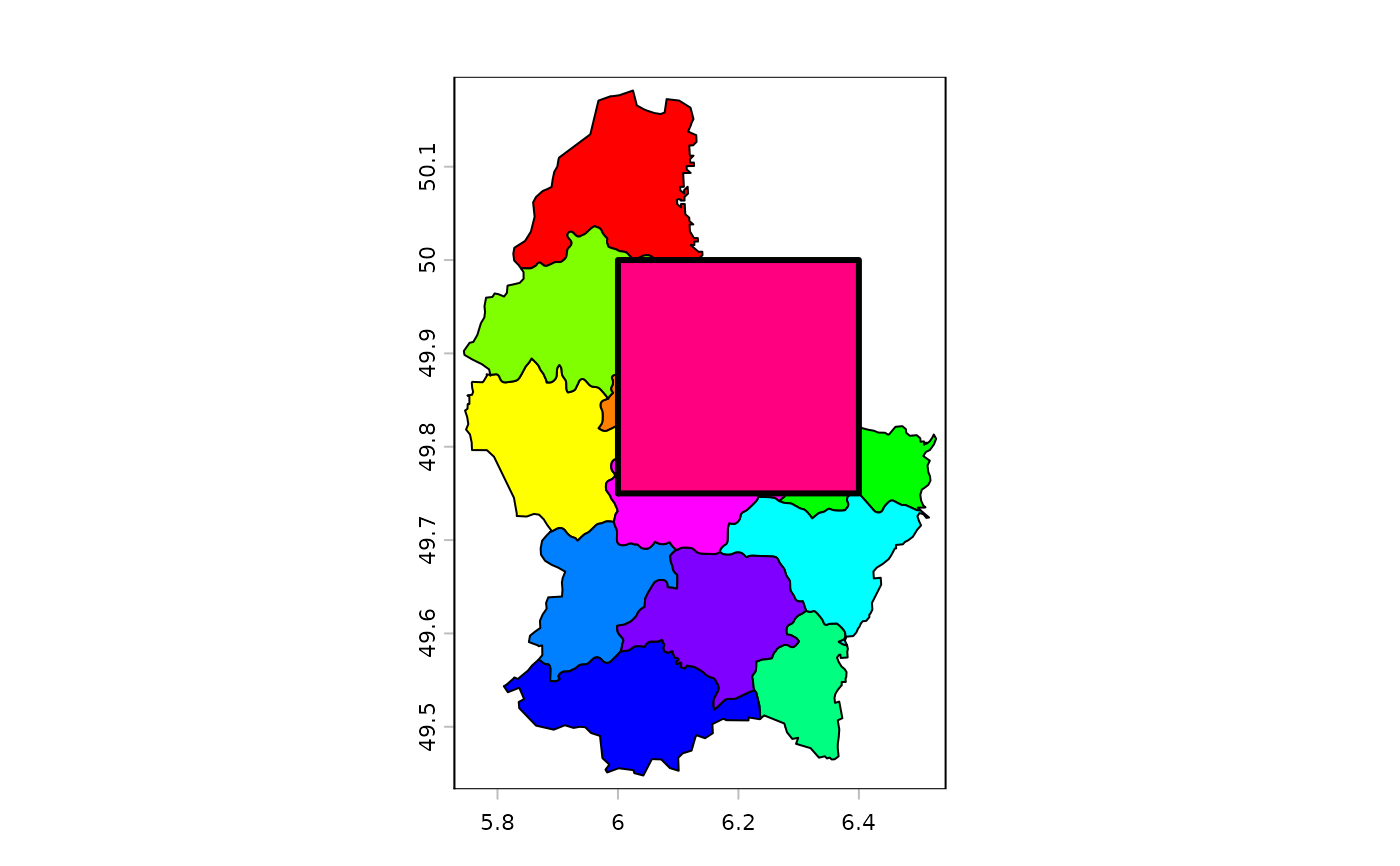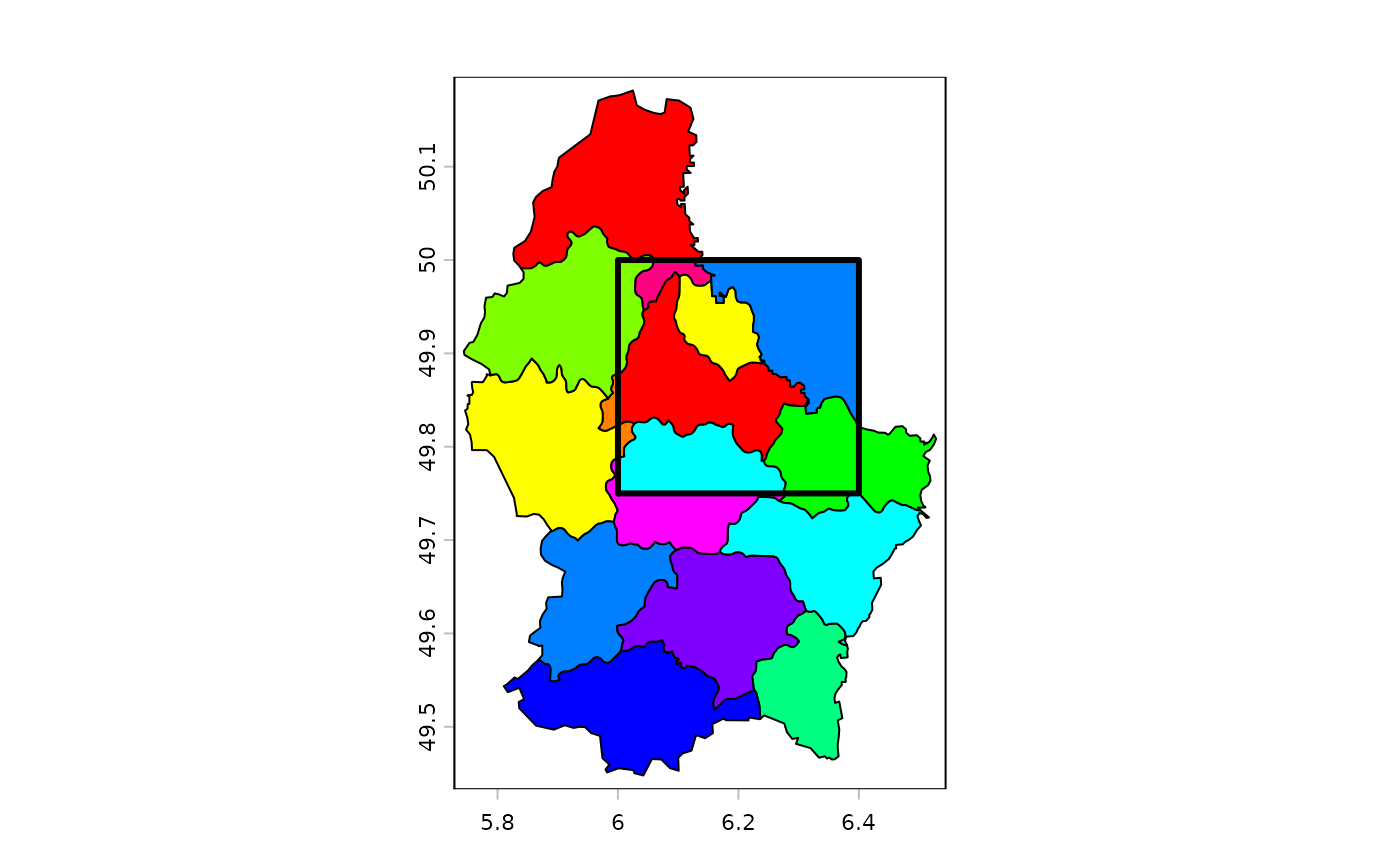Replace NA or other values in SpatRaster x with the values of SpatRaster y

For polygons: areas of x that overlap with y are replaced by y or, if identity=TRUE intersected with y.

# S4 method for SpatRaster,SpatRaster
cover(x, y, values=NA, filename="", ...)

# S4 method for SpatVector,SpatVector
cover(x, y, identity=FALSE, expand=TRUE)

## Arguments

x

SpatRaster or SpatVector

y

Same as x

values

numeric. The cell values in x to be replaced by the values in y

filename

character. Output filename

...

additional arguments for writing files as in writeRaster

identity

logical. If TRUE overlapping areas are intersected rather than replaced

expand

logical. Should parts of y that are outside of x be included?

SpatRaster

## Examples

r1 <- r2 <- rast(ncols=36, nrows=18)
values(r1) <- 1:ncell(r1)
values(r2) <- runif(ncell(r2))
r2 <- classify(r2, cbind(-Inf, 0.5, NA))
r3 <- cover(r2, r1)

p <- vect(system.file("ex/lux.shp", package="terra"))
e <- as.polygons(ext(6, 6.4, 49.75, 50))
values(e) <- data.frame(y=10)

cv <- cover(p, e)
plot(cv, col=rainbow(12))
ci <- cover(p, e, identity=TRUE)
lines(e, lwd=3)plot(ci, col=rainbow(12))
lines(e, lwd=3)